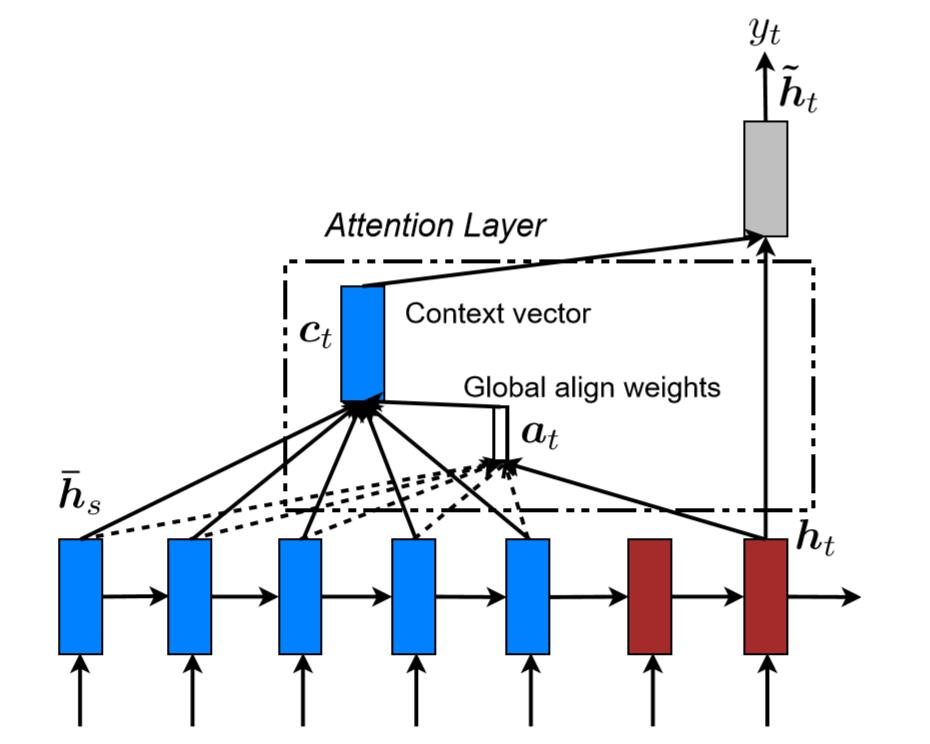# Attention论文列表

• Sequence to Sequence Learning with Neural Networks, 2014. 机器翻译(Machine Translation)领域论文，使用两个RNN进行机器翻译，亮点在于将source sentence做了逆向输入。未看
• Neural Machine Translation by Jointly Learning to Align and Translation, 2015. 同样属于MT领域，作者认为将source sentence由Encoder映射成一个向量，无法突破翻译瓶颈，并提出了动态搜索relevant part of source sentence， 即Attention思想。未看
• Effective Approaches to Attention-based Neural Machine Translation, 2015. 论文提出了global attentionlocal attention, 博文对其做了比较详细的解释，我也会在下面详细介绍。
• Attention is All You Need, 2017. self-attention机制及Transformer模型。
• Pointer Sentinel Mixture Models, 2016. cs224n课程曾提到过的，当时并没理解。李宏毅老师的课程视频也有讲解。以往的RNN模型虽然对输入序列长度适应，但输出的序列仍然属于固定的集合。Pointer模型能从输入序列中学习输出单词，从而解决模型无法输出未学习到的单词情况。
• Listen, Attend and Spell, 2015. 李宏毅老师曾调侃Attenion模型一定要以三个动词开始。

# Attention模型之一详解

“attention”产生于机器翻译领域，其含义是允许模型学习不同任务间的对齐(learn alignments between different modalities)，比如图片描述生成，语音识别。神经机器翻译(NMT)相比于传统MT,占用内存小，decoder实现简单以及end-to-end。

NMT系统是神经网络模型在条件概率$p(y|x)$下将source sentence，$x_1,…,x_n$ 翻译成target sentence, $y_1,…,y_n$,的过程。NMT的一般由Encoder和Decoder，前者学习source sentence的向量化表示，后者是每次生成一个目标单词，故条件概率为：
$$\log p(y|x) = \sum_{j=1}^m \log p(y_j|y_{<j}, \mathbf{s})$$
$\mathbf{s}$ 表示source sentence。encoder和decoder一般使用RNN(GRU/LSTM)来实现。对于预测单词$y_j$，其概率计算可归纳为：
$$p(y_j|y_{<j},\mathbf{s}) = \text{softmax}(g(\textbf{h}_j))$$

softmax是在整个词汇表长度上进行的，$\mathbf{h}_j$是RNN的单元，其计算可归纳为：
$$\mathbf{h_j} = f(h_{j-1},\mathbf{s})$$

$f$可以表示普通RNN单元、GRU或LSTM计算实现。

## Global Attention

$$\text{score}(\mathbf{h}_t,\bar{\mathbf{h}_s}) = \begin{cases} \mathbf{h}_t^T \bar{\mathbf{h}_s} \qquad\qquad\qquad dot \\ \mathbf{h}_t^T \mathbf{W}_a \bar{\mathbf{h}_s} \qquad\qquad general \\ \mathbf{v}_a^T \text{tanh}[\mathbf{h}_t^T : \bar{\mathbf{h}_s}] \quad concat \\ \end{cases}$$

\mathbf{a}_t(\mathbf{s}) = \text{align}(\mathbf{h_t}, \bar{\mathbf{h}}_s)

$$=\frac{\exp(\text{score}(\mathbf{h}_t^T,\bar{\mathbf{h}_s}))}{ \sum_s \exp(\mathbf{h}_t^T,\bar{\mathbf{h}_s})}$$

$$c_t = \sum a_t(s)\cdot\bar{h}_s$$

$$\tilde{\mathbf{h}}_t = \text{tanh}(\mathbf{W}_c[c_t;\mathbf{h}_t])$$

$$p(y_t|y_{<t}, x) = \text{softmax}(\mathbf{W}_s \tilde{\mathbf{h}_t})$$

Attention层中的$W_a,W_c$是超参数，通过训练学习。$h_t$与$a_t$的连线为实线，因为对于每一个 $\bar{h}_s$的权重计算都需要使用 $h_t$。而 $\bar{h}_s$是逐个计算的，因此是虚线。$a_t$经过softmax后，与每个source hidden state相乘得到 $c_t$。$c_t$与 $h_t$相乘得到 $\tilde{h}_t$。

## Local Attention

Local Attention是受soft/hard attention启发。其思想是当序列过长的时候，将注意力分散到全部的source hidden state并不合适，计算量很大。因此，想缩小attention的范围。根据经验设置一个超参数$D$，对于每次预测的time-step t选择一个position $p_t$。然后在 $[p_t-D , p_t+D]$窗口范围进行Attention。$p_t$的选取有两种：

• Monotonic alignment (local-m)：$p_t = t$
• Predictive alignment (local-p)：$p_t = S \cdot \text{sigmoid}(v_p^T\text{tanh}(W_p\mathbf{h}_t))$ 当然$a_t(s)$函数也有变动，可详见论文。

## 总结# Attention模型之二详解

BERT模型中的QKV概念

Attention和Transformer